Courses

# NCERT Solutions - Number System, Mathematics, Class 9 Class 9 Notes | EduRev

## Class 9 : NCERT Solutions - Number System, Mathematics, Class 9 Class 9 Notes | EduRev

The document NCERT Solutions - Number System, Mathematics, Class 9 Class 9 Notes | EduRev is a part of Class 9 category.
All you need of Class 9 at this link: Class 9

Question 1. Is zero a rational number? Can you write it in the form p/q  , where p and q are integers and q ≠ 0?

Solution: Yes, zero is a rational number. We can write it in the form p/q.
That is zero =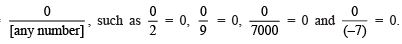Question 2. Find six rational numbers between 3 and 4.

Solution: We know that there are an infinite number of rational numbers between two rational numbers. Therefore, six rational numbers between 3 and 4 can be: Let x = 3 and y = 4, also n = 6

∴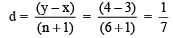Since, the six rational numbers between x and y are:

(x + d), (x + 2d), (x + 3d), (x + 4d), (x + 5d) and (x + 6d).

∴  The six rational numbers between 3 and 4 are: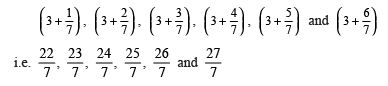Question 3. Find five rational numbers between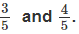.

Solution: Let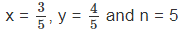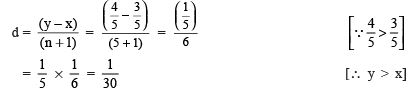∵ The five rational numbers between ‘x’ and ‘y’ are:

(x + d); (x + 2d); (x + 3d); (x + 4d) and (x + 5d).

∴ Five rational numbers between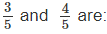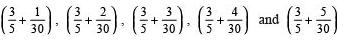or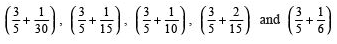or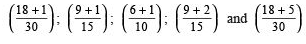or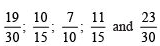or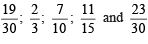Another method

∴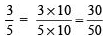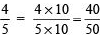∴ Five rational numbers between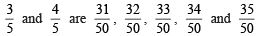Question 4. State whether the following statements are true or false. Give reasons for your answers.

(i) Every natural number is a whole number.

(ii) Every integer is a whole number.

(iii) Every rational number is a whole number.

Solution: (i) True statement [∵ The collection of all natural numbers and 0 is called whole numbers]

(ii) False statement [∵ Integers such as –1, –2 are non-whole numbers]

(iii) False statement [∵ Rational number 1/2 is not a whole number]

Question 1. State whether the following statements are true or false. Justify your answers.
(i) Every irrational number is a real number.
(ii) Every point on the number line is of the form
√m , where m is a natural number.
(iii) Every real number is an irrational number.
Solution:
(i) True statement, because all rational numbers and all irrational numbers form the group (collection) of real numbers.

(ii) False statement, because no negative number can be the square root of any natural number.

(iii) False statement, because rational numbers are also a part of real numbers.

Question 2. Are the square roots of all positive integers irrational? If not, give an example of the square root of a number that is a rational number.

Solution: No, if we take a positive integer, say 4 its square root is 2, which is a rational number.

REMEMBER

According to the Pythagoras theorem, in a right-angled triangle, the square of the hypotenuse is equal to the sum of the squares of the other two sides.
In the figure: OB2 =OA2 + AB2
ﬁ OB2 =12 + 12 = 2 ⇒ OB =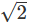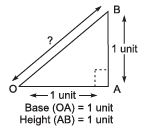Question 3: Show how 5 can be represented on the number line.

Solution: Let us take the horizontal line XOX" as the x-axis. Mark O as its origin such that it represents 0.
Cut off OA = 1 unit, AB = 1 unit.
∴ OB = 2 units Draw a perpendicular BC ⊥ OX.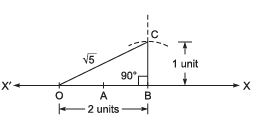Cut off BC = 1 unit.
Since OBC is a right triangle.
∴ OB2 + OC2 = OC2
or 22 + 12 = OC2 or 4 + 1 = OC2 or OC2 = 5 ⇒
OC = √5
With O as centre and OC as radius, draw an arc intersecting OX at D.
Since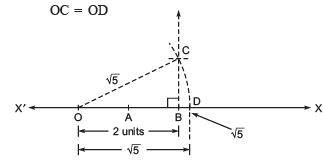∴ OD represents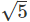on XOX".

Question 1. Write the following in decimal form and say what kind of decimal expansion each has:Solution: (i) We have 36/100 = 0.36
∴ The decimal expansion of 36/100 is terminating.

(ii) Dividing 1 by 11, we have: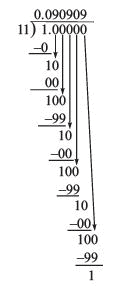∴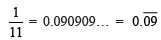Thus, the decimal expansion is “non-terminating repeating”.
Note: The bar above the digits indicates the block of digits that repeats. Here, the repeating block is 09.

(iii) To write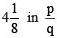form, we have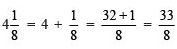Now, dividing 33 by 8, we have: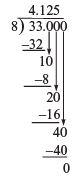Remainder = 0, means the process of division terminates.

∴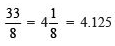Thus, the decimal expansion is terminating.

( iv) Dividing 3 by 13, we have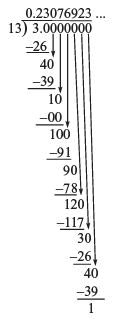Here, the repeating block of digits is 230769.

∴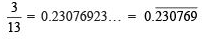Thus, the decimal expansion of 3/13  is “non-terminating repeating”.

( v ) Dividing 2 by 11, we have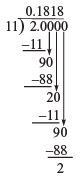Here, the repeating block of digits is 18.

∴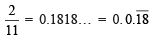Thus, the decimal expansion of 2/11  is “non-terminating repeating”.

(vi) Dividing 329 by 400, we have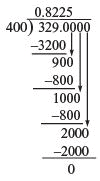Remainder = 0, means the process of division terminates.

∴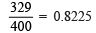Thus, the decimal expansion of 329/400  is terminating.

Question 2. You know that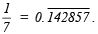Can you predict what the decimal expansions of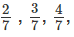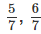are, without actually doing the long division? If so, how?
Solution:
We are given that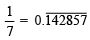∴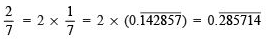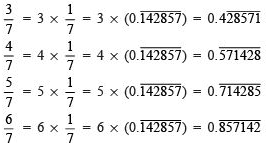Thus, without actually doing the long division we can predict the decimal expansions of the above given rational numbers.

Question 3. Express the following in the form p/q , where p and q are integers and q ≠ 0.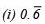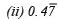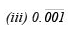Solution: (i) Let x =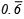= 0.6666… Since, there is one repeating digit.

∴  We multiply both sides by 10,
10x = (0.666…) x 10
or 10x = 6.6666…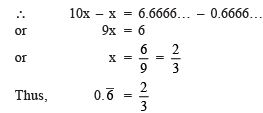(ii) Let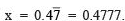∴ = 10 x (0.4777...)
or    lOx = 4.777    ...(1)
and    lOOx = 47.777    ...(2)
Subtracting (1) from (2), we have
100x - 10x = (47.777...) - (4.777...)
90x = 43

or x = 43/90
Thus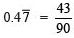(iii) Let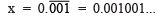.. ...(1)

Here, we have three repeating digits after the decimal point, therefore we multiply by 1000.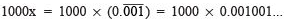or  1000x    =    1.001001...    ...(2)
Subtracting (1) from (2), we have
1000x - x    =    (1.001...) -    (0.001...)
or    999x    =    1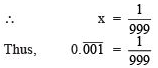Question 4. Express 0.99999… in the form p q . Are you surprised by your answer? With your teacher and classmates discuss why the answer makes sense.
Solution:
Let x = 0.99999... ...(1)
Multiply both sides by 10,
we have [∵ There is only one repeating digit.]
10 x x = 10 x (0.99999…)
or 10x = 9.9999 ...(2)
Subtracting (1) from (2),
we get 10x – x = (9.9999…) – (0.9999…)
or 9x = 9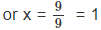Thus, 0.9999… = 1 As 0.9999… goes on forever, there is no gap between 1 and 0.9999…

Hence both are equal.

Question 5. What can the maximum number of digits be in the repeating block of digits in the decimal expansion of 1/17? Perform the division to check your answer.
Solution:
Since, the number of entries in the repeating block of digits is less than the divisor.
In 1/17 , the divisor is 17.

∴    The maximum number of digits in the repeating block is 16. To perform the long division, we have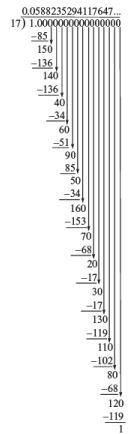The remainder 1 is the same digit from which we started the division.

∴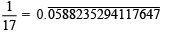Thus, there are 16 digits in the repeating block in the decimal expansion of 1/17. Hence, our answer is verified.

Question 6. Look at several examples of rational numbers in the form p/q (q ≠ 0), where p and qare integers with no common factors other than 1 and having terminating decimal representations (expansions). Can you guess what property q must satisfy?
Solution:
Let us look at decimal expansion of the following terminating rational numbers: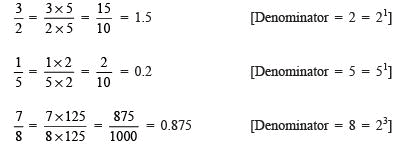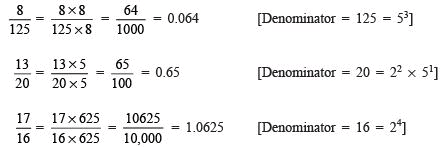We observe that the prime factorisation of q (i.e. denominator) has only powers of 2 or powers of 5 or powers of both.

 Note: If the denominator of a rational number (in its standard form) has prime factors either 2 or 5 or both, then and only then it can be represented as a terminating decimal.

Question 7. Write three numbers whose decimal expansions are non-terminating non-recurring.

Solution: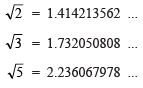Question 8. Find three different irrational numbers between the rational numbers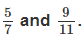.

Solution: To express decimal expansion ofwe have:

∴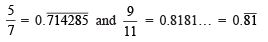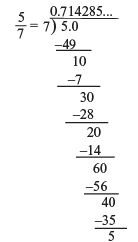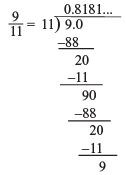As there are an infinite number of irrational numbers between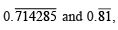, any three of them can be:
(i) 0.750750075000750…
(ii) 0.767076700767000767…
(iii) 0.78080078008000780…

Offer running on EduRev: Apply code STAYHOME200 to get INR 200 off on our premium plan EduRev Infinity!

,

,

,

,

,

,

,

,

,

,

,

,

,

,

,

,

,

,

,

,

,

,

,

,

,

,

,

;